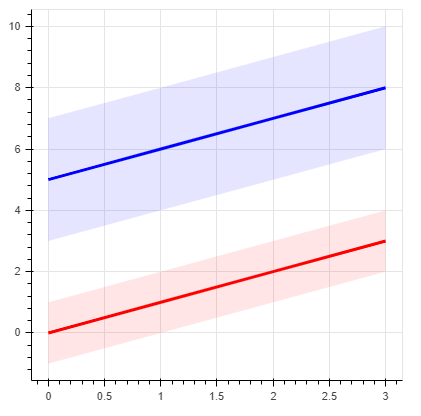# Linking multiple hover_glyphs?

Hi, I am plotting a series of lines, each with a “varea” surrounding them to represent uncertainty. I would like to make it such that the varea triggers its hover properties (e.g. hover_fill_alpha) when the corresponding line is hovered over, not when the varea itself is hovered over.

So I’m wondering if there is a way to link the hover action of a line renderer to the hover action of a varea renderer?

In the code below, I have limited the hovertool to the line renderers so that the varea won’t trigger when the varea alone is hovered over, and I was hoping something like
“lineList.hover_glyph.js_link(‘visible’, areaList.hover_glyph, ‘visible’)”
would work. But I see that the visible property is linked to the renderer itself, not the glyphs within, so no such ‘visible’ property exists. Is there a property associated with the hover_glyph that does indicate when it has been activated that I could use to trigger the varea’s hover_glyph?

Here is some simple example code that shows the desired setup, minus the varea hover_fill_alpha triggering:

``````from bokeh.io import output_file, output_notebook
from bokeh.plotting import figure, show
from bokeh.models import HoverTool

fig = figure(plot_width=400, plot_height=400, tools="", toolbar_location=None)

x = np.array([[0, 1, 2, 3], [0, 1, 2, 3]])
y = np.array([[0, 1, 2, 3], [5, 6, 7, 8]])
dy = np.array([[1, 1, 1, 1], [2, 2, 2, 2]])
color = ['red', 'blue']

lineList = []
areaList = []

for i in range(2):
activeLine = fig.line(x=x[i], y=y[i],
color=color[i], line_width=3, hover_line_width = 5)
lineList.append(activeLine)

activeArea = fig.varea(x[i],
y[i] + dy[i],
y[i] - dy[i],
fill_color=color[i], fill_alpha=0.1, hover_fill_alpha = 0.3)
areaList.append(activeArea)

fig.add_tools(HoverTool(
tooltips=[("x", "@x{0.0}"), ("y", "@y{0,0.0}")],
renderers = lineList
))

show(fig)
``````Thanks for any help!